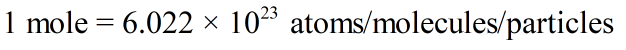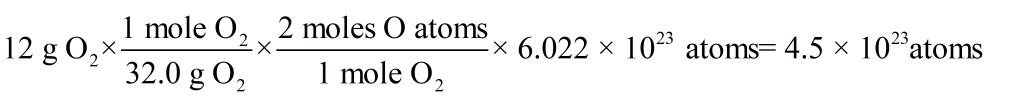Stoichiometry homework Help at TutorEye

## Top Questions

erature and pressure? Record your answer in scientific notation using 3 significant figures.
View More

## Stoichiometry

• Stoichiometry is derived from greek words, “Stoicheion'' means element and'' metron” means measure. Stoichiometry is the study of the quantitative aspects of a chemical change.

• Stoichiometry is the calculation of the quantities of reactants and products involved in a chemical reaction. In stoichiometry we try to find the masses, volumes, number of particles, energies etc.. of reactants taking part in a chemical reaction and products formed.

• In stoichiometry we always use a balanced chemical equation because an unbalanced chemical equation violates the Law of “conservation of mass”.

• Some basic relations are:

1 mole of a gas = GMW of a gas = 22.4 L at STP

1 mole of a substance = GMW

• For Homo atomic molecules GMW = Atomicity x GAW

• For Hetero atomic molecules GMW = sum of Gram atomic weight of all constituents.It is based on the chemical equation and on the relationship between mass and moles.

• The Avogadro number can be used to calculate the number of atoms or molecules in the given moles of the substances. The expression can be write as:## Stoichiometry Questions

Question 1: Calculate the number of atoms in 12 g of oxygen gas.

Solution:

Molar mass of oxygen gas = 32.0 g/mol

Number of atoms in 1 oxygen gas molecule = 2Question  2: Stoichiometry is derived from greek words, “Stoicheion” means element and“ metron” means _________.

(a) Compound

(b) Calculation

(c) Value

(d) Measure

Solution : Stoichiometry is derived from greek words, “Stoicheion” means element and“ metron” means measure

Question 3: 1 mole of any gas   at STP occupies ___________.

(a) 32 litre

(b) 44 litre

(c) 22.4 litre

(d) All the above

Solution : 1 mole of any gas at STP occupies 22.4 litres.

### How do you calculate stoichiometry?

There are some guidelines to calculate stoichiometry.

Assign 1 as the coefficient for the most complex species.

Balance the single-element species in last.

Eliminate fractional coefficients.

While balancing add coefficients only, do not change the chemical formula of any compound.

The number of atoms should be the same on both sides of the reaction.

For example- Let’s balance this equation using guidelinesThe equation is not balanced as the number of N and O atoms is more on left (reactants) side

Assigning 1 coefficient in front of the most complex speciesBalancing single element compounds### What is stoichiometry in chemistry?

Stoichiometry is the quantitative relationship between the number of moles of different reactants and products in a chemical reaction. We know that a chemical equation must be balanced and must have the same number of different atoms in the reactants and products. To balance a chemical equation we add coefficients in front of reactants and products. These coefficients are known as stoichiometric coefficients and their study is called stoichiometry.

### Why is stoichiometry so hard?

Stoichiometry problems can have multiple steps, which is not easy to memorize. Basic mathematics skills are required for stoichiometric calculations. Not understanding concepts can make it even more difficult. However, practicing solving problems after learning the concept well makes stoichiometry a lot more fun and interesting.

### How do you do stoichiometry for dummies?

There are four simple steps to calculate stoichiometry:

• Balance the equation.

• Convert the units of given substance to moles.

• Use mole ratio and calculate the moles of substance yielded in the reaction.

• Convert the moles of wanted substance to desired units.